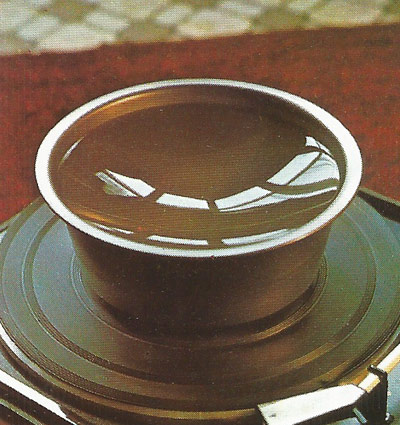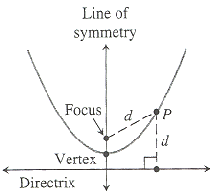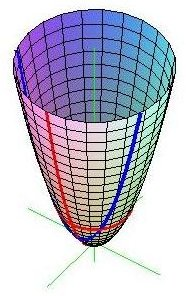A# parabolaFigure 4. The surface of a rotating liquid is a paraboloid.

The parabola is a curve, with an eccentricity equal to 1, obtained by slicing a cone with a plane parallel to one side of the cone. It is one of the four types of conic section. A parabola can also be considered to be an ellipse with an infinite major axis.

One of the most studied curves in the history of mathematics, a parabola is the outline of the figure obtained if a right circular cone is cut by a plane that is exactly parallel to the cone's side. Just as the circle is a limiting case of the ellipse when the two foci coincide, the parabola is a limiting case of the ellipse when one of the foci is moved to infinity. As the French mathematician Henri Fabre eloquently put it, a parabola is an ellipse that "seeks in vain for its second, lost center." Johannes Kepler drew this further connection between the parabola and other conic sections:

Because of its intermediate nature the parabola occupies a middle position [between the ellipse and the hyperbola]. As it is produced it does not spread out its arms like the hyperbola but contracts them and brings them nearer to parallel, always encompassing more, yet always striving for less – whereas the hyperbola, the more it encompasses, the more it tries to obtain.Figure 1. The parabola

A parabola is the locus of all points in a plane that are equidistant from a given line, known as the directrix, and a given point not on the line, known as the focus (see Figure 1). The Cartesian equation of a parabola that opens upward and has its vertex (turning point) at the origin is y = 4ax2, where a is the distance from the vertex to the focus, and the quantity 4a is known as the latus rectum. More generally, any quadratic equation of the form y = ax2 + bx + c, where a is not zero, graphs a parabola. The simplest form of this, when a = 1, and both b and c are zero, is y = x2. Like the circle, but unlike the ellipse and the hyperbola, the parabola has only one distinct shape. In other words, any parabola can be superimposed exactly on any other parabola simply by rotating, translating (sliding), and/or enlarging or shrinking it.

Euclid dealt with the parabola in his Conic Sections, and, although this treatise was lost, it provided a foundation for the first four books by Apollonius of the same name. Galileo discovered that a cannonball, or any other projectile launched at an angle to ground, follows a parabolic path: a result that immediately grabbed the attention not only scientists but of monarchs and military leaders. René Descartes, in writing La Géométrie (1637), chose the parabola to illustrate his innovative analytical geometry. In 1992, Rudolph Marcus of the California Institute of Technology won the Nobel Prize in Chemistry for his work showing that parabolic reaction surfaces can be used to calculate how fast electrons travel in molecules. His most famous theoretical result, an inverted rate-energy parabola, predicts electron transfer will slow down at very high reaction free energies.

## Dürer's shell curveFigure 2. Dürer's shell curve

Dürer's shell curve may be defined as follows: given a parabola and a line that is tangent to the parabola, it is the glissette of a point on a line sliding between the parabola and the tangent. It has the equation

(x2 + xy + ax - b2) = (b2 - x2)(x - y + a)2.

## ParaboloidFigure 3. The paraboloid

A paraboloid is the surface of revolution of a parabola (see Figure 3). It is a quadratic surface described by the equation z = a(x2 + y2).

A uniformly rotating liquid acquires a paraboloidal surface (see Figure 4) from the interaction of gravity and centrifugal forces, as can be seen by spinning a pan of liquid on a turntable. This surface is perfect for radio- and optical-telescope mirrors; a mirror surface can be made by spinning plastic resin in a dish while it sets and coating it with a metal.